### Adding a Slope node

This node creates a terrain plane with an editable inclination and orientation either by specifying the minimum and maximum height values or by adjusting the slope angle.

To add a Slope node, right-click in the Graph Editor and select Create Node  > Terrain Generation  > Slope.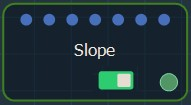Double click on the node to open its parameters: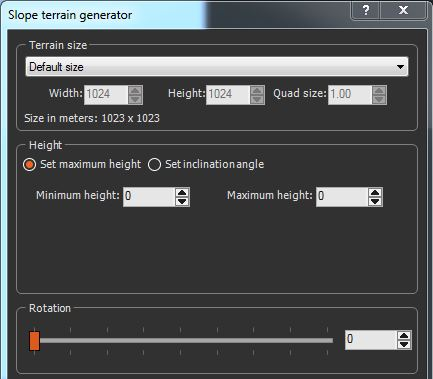### Setting the terrain size

• To change the terrain size, edit the Width and Height fields in number of vertices or use a preset size from the list.
• To change the quad size, edit the Quad size field in meters.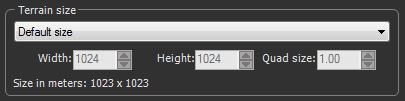### Setting the slope height

Two ways exist to set the slope of the terrain:

• Click Set maximum height to define the slope with its minimum and maximum heights.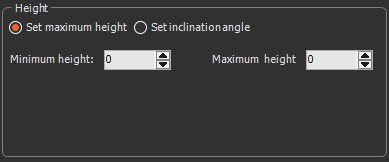Set the Minimum Heights and Maximum Heights of the slope to determine the lowest and highest vertices, respectively. If the values in both boxes are the same, a constant is created.

• Click Set inclination angle to set the minimum height and inclination angle. This parameter allows the orientation of the slope to be adjusted easily.
• Use the Inclination angle slider to set the angle from 0 to 85 degrees between the plane and the horizontal.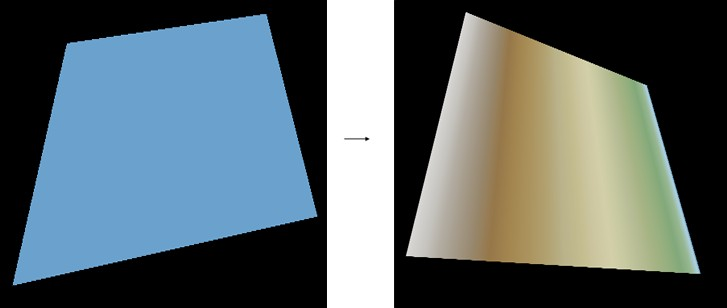Moving the slider left flattens the terrain towards the horizontal plane; moving the slider to the right moves the terrain towards the vertical plane.

• Set the Minimum height to define the lowest vertices of the terrain.

### Setting the slope rotation

Use the Rotation slider to set the slope rotation.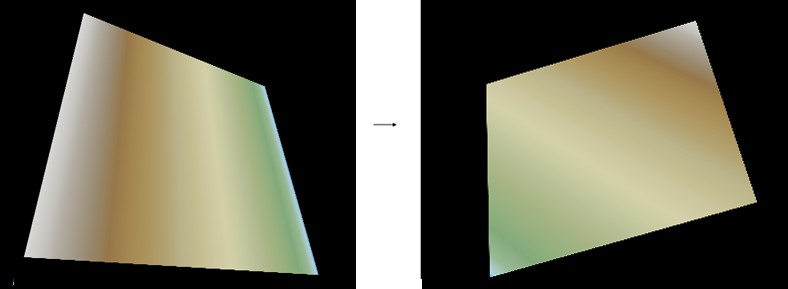The terrain rotates 360 degrees around the center.

### Parameters

Parameter Use
Terrain size See "Part common to all generator nodes"
Set maximum height The plane will be specified with its minimum and maximum heights
Minimum height Determines the height of the lowest vertices of the terrain
Maximum height Determines the height of the highest vertices of the terrain
Set inclination angle
Minimum height Determines the height of the lowest vertices of the terrain
Inclination angle Determines the angle between the plane and the horizontal
Rotation Rotation angle

Copyright © 2021 · All Rights Reserved · Wysilab